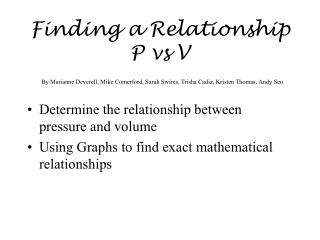DownloadDownload PresentationDetermine the relationship between pressure and volume

# Determine the relationship between pressure and volume

Télécharger la présentation## Determine the relationship between pressure and volume

- - - - - - - - - - - - - - - - - - - - - - - - - - - E N D - - - - - - - - - - - - - - - - - - - - - - - - - - -
##### Presentation Transcript

1. Finding a Relationship P vs VBy Marianne Deverell, Mike Comerford, Sarah Swires, Trisha Cadiz, Kristen Thomas, Andy Seo • Determine the relationship between pressure and volume • Using Graphs to find exact mathematical relationships

2. Science What is volume and Pressure Have worked with gasses and compressibility of gasses Math Being able to use Logger Pro Application Ability to use graphs to find mathematical relationships What we Gotta Know:

3. Procedure • Use gas sensor probes to collect the data for pressure at different volumes

4. Use this information to determine if a relationship exists between volume and pressure • After deciding if there is a relationship, show with a correlative line that, there exists a relationship.

5. Where we can use this • In science, we could use this as an exploratory tool in a first year chemistry class. This could introduce the topic of Boyle’s Law • In a math class, this could be used as an extension of mathematical statistics or in an advanced algebra class.